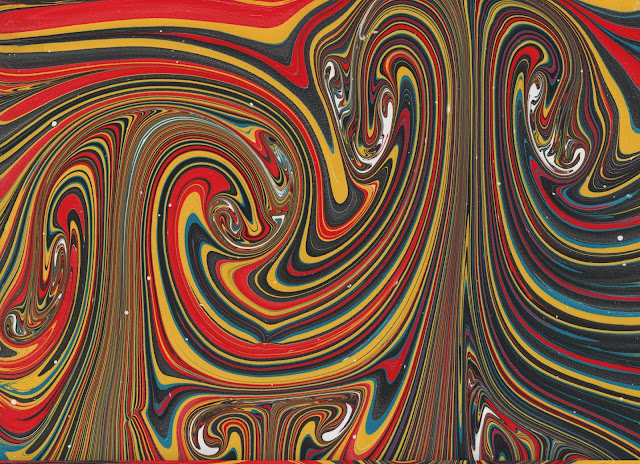# Voluntocracy

Governance by those who do the work.

## Friday, July 27, 2018

### Bubbles in MarblingBubbles and parted paints
The St.Johns marbling technique uses stylus speeds of only a few centimeters per second. When a stylus is moved quickly through the tank fluid, air bubbles are formed. Bubbles were formed drawing a 25 mm diameter dowel faster than (V=) 20 cm/s through the tank (which also parted the floating paints) in my previous post.

While the formation of bubbles could be due to non-Newtonian fluid behavior, it is worth examining the conditions assuming a Newtonian fluid. A 25 mm diameter dowel submerged 12.5 mm will behave more like half of a 25 mm diameter sphere than a cylinder. If the kinematic viscosity (ν=0.001 m2/s) of the liquid is 1000 times that of water, then the Reynolds number is about 5, far less than the 90 needed to spawn vortexes. Re is inversely proportional to viscosity; reducing the kinematic viscosity by a factor of 10 raises Re to 50.

A half sphere of diameter d has buoyant-pressure (restoring force divided by cross-section area) of about 81 N/m2. Surface tension pressure (restoring force divided by cross-section area) of water is roughly 3.7 N/m2.

Drag is the force on the object moving through the tank fluid. There must be an equal and opposite net force on the liquid. Drag D for a sphere is the product of the friction coefficient CD, frontal area (π/4*d2), and dynamic head V2*ρ/2 (for water ρ=997). That force divided by the frontal area of the object is a pressure (suction actually).

A bubble will be formed if this suction behind the moving stylus is larger than the sum of the restoring forces at the liquid surface.

For ν=1000 mm2/s (1000 times that of water) the suction behind our 25 mm diameter dowel is 88 N/m2, which exceeds the restoring pressures 81 N/m2 and 3.7 N/m2, and bubbles can result.

The slower motions and smaller styluses that the St.Johns usually use have Reynolds numbers much smaller than 5. Thus the marblings they create don't evidence inertial effects (versus the mushroom designs of my previous post)."Mushroom" flow from straight strokes
Kinematic viscosities below 50 mm2/s (50 times that of water) would be needed to spawn vortexes in marbling.  At 50 mm2/s viscosity, a 25 mm cylinder would need to be moved at 20 cm/s over at least 16 cm before the first vortex was shed.

In water, a 5 mm cylinder moving at 2 cm/s would shed vortexes 3 cm apart.   A 1 mm diameter stylus moved in a straight path at 5 cm/s would not shed vortexes.

So existing evidence of Karman (shed) vortexes is only likely to be found in marbling produced on a tank filled with water.